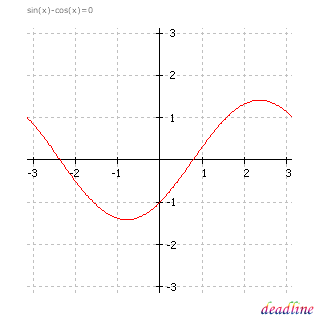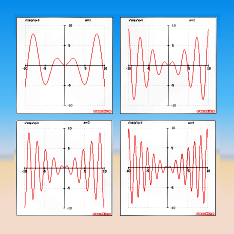# How to plot graphs

## Evaluating functions and derivatives

Calculate the value of a function and the value of the first two derivatives in an x-point.

You can type complicated expressions that include trigonometric functions, hyperbolic or exponential functions and they will be evaluated instantly and accurately.

Tip. A downsized version of this feature can be found in any project window. You can evaluate the current function and the first derivative in any point from the current interval by clicking inside the graph.

## Equation solving

Solve equations graphically and numerically.

DeadLine automatically displays all the real roots of the equation in a scrollable list. If you want to see a root on the graph, select it in the list.Now you can change the interval, change the precision of the graph and save the results.

## Plot graphs

• Zoom in / zoom out to narrow / widen the scope, while remaining centered.
• Click outside the graph, to move the graph in one of the eight directions, indicated by a different mouse cursor.
• Move the cursor inside the graph to evaluate the function and the first derivative in any point from the current domain.
• Press F9, and customize the graph. Change the current interval by explicitly adjusting the bounds.

## How to adjust the precision of the graph

• Press F9 or click the Options button.
• Type the number of points in Graph points, which should be an integer value from 10 to 1000 (the default value is 200, the bigger is the precision, the more accurate is the graph).
• Graph changes will take place instantly.

## Plot graphs in full-screen view

• Full-screen view lets you explore the graph in a resizable window.
• When you enter the full-screen mode, the project window is hidden.
• To use the zoom features or to move the graph, take advantage of the keyboard shortcuts.
• If you want to restore the normal view, press F11 or Esc.

## How to save graphs

• Select Graph/Save graph from the menu.
• Enter the name of the file. You can save the graph in one of the following formats: GIF, PNG, BMP or TIFF. Read more about choosing the right format.
• The default location for saved graphs, as well as for saved roots and extrema, is: My Documents/DeadLine Results

## Plot parametric graphs

• Define the equation name and the initial values of the parameters.
• Edit the parameters and then see the influence on the graph.
• Take a look at four graphs for x sin(a*x) that illustrate this.
Download DeadLine, the interactive equation solver. Plot graphs, retrieve equation roots, and find derivatives in an intuitive way.
DeadLine OnLine - free equation solver. Copyright 2003-2007 Ionut Alex. Chitu. | Contact | Sitemap | Google Operating System